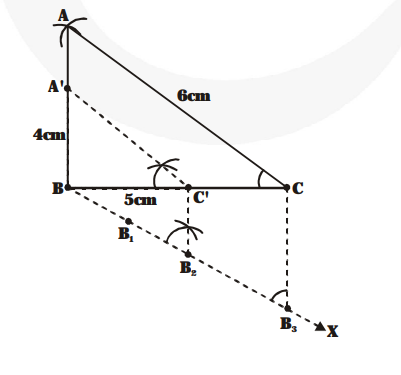# Construct a triangle of sides 4 cm,

Question:

Construct a triangle of sides 4 cm, 5 cm and 6 cm and then a triangle similar to it whose sides are 2/3 of the correspoding sides of the first triangle.

Solution:

Steps of construction :1. Construct ABC, such that AB = 4 cm,

BC = 5 cm and CA = 6 cm.

2. Draw any ray BX making an acute angle with BC (below the side BC).

3. Mark three points $\mathrm{B}_{1}, \mathrm{~B}_{2}, \mathrm{~B}_{3}$ on $\mathrm{BX}$ such that

$\mathrm{BB}_{1}=\mathrm{B}_{1} \mathrm{~B}_{2}=\mathrm{B}_{2} \mathrm{~B}_{3} .$

4. Join $\mathrm{B}_{3} \mathrm{C}$

5. Through $\mathrm{B}$ draw $\mathrm{B} \mathrm{C}^{\prime} \| \mathrm{B}, \mathrm{C}$ and let it intersect

$\mathrm{BC}$ at $\mathrm{C}^{\prime}$.

6. Through $\mathrm{C}^{\prime}$ draw $\mathrm{C}^{\prime} \mathrm{A}^{\prime} \| \mathrm{CA}$ and let it intersect

BA at $\mathrm{A}^{\prime}$.

$\triangle \mathrm{A}^{\prime} \mathrm{BC}^{\prime}$ is the required triangle similar to the given $\triangle \mathrm{ABC}$ whose side are $2 / 3$ of the corresponding sides of the $\triangle \mathrm{ABC}$.